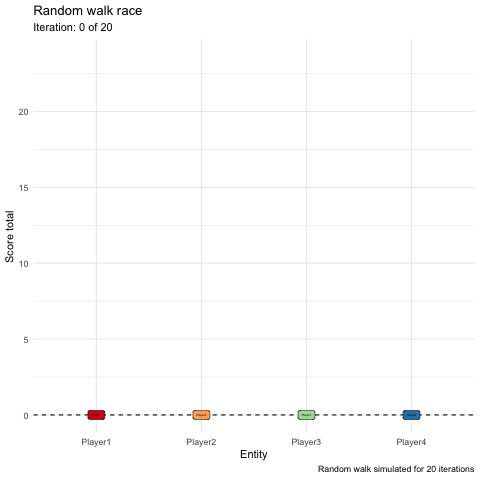# wiki: Random walk animation #148

Closed
opened this issue Aug 18, 2018 · 1 comment
Closed

# wiki: Random walk animation#148

opened this issue Aug 18, 2018 · 1 comment

###kevinsoo commented Aug 18, 2018

Using `ggplot2` and `gganimate` to visualize simple random walks, used to determine draft order in my Fantasy Football league.

# Create data

Random walks for 4 entities for a predetermined number of steps. Each step, I draw from a normal distribution with mean of 1, so that numbers are generally positive. The cumulative sum will be plotted, to show which entity gains the highest score during the walk.

``````# Load libraries
library(tidyverse)
library(gganimate)

# Generate data
n <- 20 # Number of iterations/steps

# Random walk for 4 players
df <- tibble(
ite = 0:n,
Player1 = c(0, rnorm(n, mean = 1)),
Player2 = c(0, rnorm(n, mean = 1)),
Player3 = c(0, rnorm(n, mean = 1)),
Player4 = c(0, rnorm(n, mean = 1))) %>%
gather(player, score, Player1:Player4) %>%
arrange(player, ite) %>%
group_by(player) %>%
mutate(totalScore = cumsum(score),
size = ifelse(ite == n, 6, 4)) # This is used to size the labels
``````

# Plot data

Plot uses labels to represent each entity, and becomes larger at the final step so the final order is clearly visible.

``````# Plot data
ggplot(df, aes(x = player, y = totalScore)) +
geom_hline(yintercept = 0, linetype ="dashed") +
geom_label(aes(label = player, fill = player, size = size)) +
theme_minimal() +
theme(legend.position = 'none') +
scale_fill_brewer(palette = "Spectral") +
labs(title = "Random walk race",
subtitle = "Iteration: {closest_state} of 20",
y = "Score total",
x = "Entity",
caption = "Random walk simulated for 20 iterations") +
transition_states(ite, transition_length = 1, state_length = 1, wrap = FALSE) +
ease_aes('linear') +
shadow_wake(wake_length = .1, wrap = FALSE)
``````